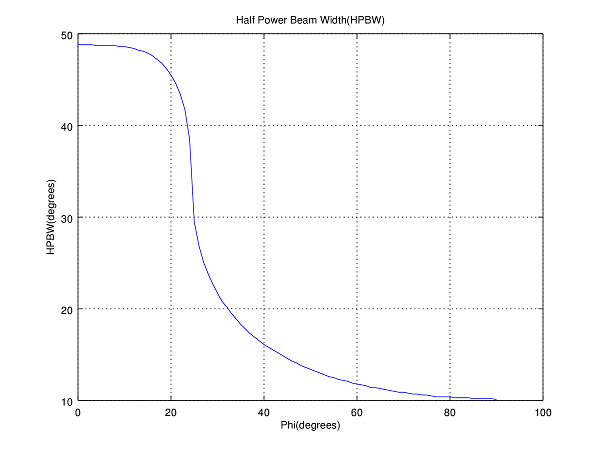# Basics of Beamforming in Wireless Communications

In the previous post we had discussed the fundamentals of a Uniform Linear Array (ULA). We had seen that as the number of array elements increases the Gain or Directivity of the array increases. We also discussed the Half Power Beam Width (HPBW) that can be approximated as 0.89×2/N radians. This is quite an accurate estimate provided that the number of array elements ‘N’ is sufficiently large.

But the max Gain is always in a direction perpendicular to the array. What if we want the array to have a high Gain in another direction such as 45 degrees. How can we achieve this? This has application in Radars where you want to search for a target by scanning over 360 degrees or in mobile communications where you want to send a signal to a particular user without causing interference to other users. One simple way is to physically rotate the antenna but that is not always a feasible solution.

Going back to the basics remember that the Electric field pattern depends upon the constructive and destructive interference of incoming waves. If we have a vector (usually called the steering vector) that aligns the rays coming in from a particular direction we would get a high Gain in that direction. Similarly we can steer a null in a particular direction if we want to reject a particular signal. This we will discuss in a future post.

MATLAB CODE

```%%%%%%%%%%%%%%%%%%%%%%%%%%%%%%%%%%%%
% BEAMFORMING USING A
% UNIFORM LINEAR ARRRAY
% COPYRIGHT RAYMAPS (C) 2018
%%%%%%%%%%%%%%%%%%%%%%%%%%%%%%%%%%%%

clear all
close all

f=1e9;
c=3e8;
l=c/f;
d=l/2;
no_elements=10;
phi=pi/6;

theta=0:pi/180:2*pi;
n=1:no_elements;
n=transpose(n);

X=exp(-i*(n-1)*2*pi*d*cos(theta)/l);
w=exp( i*(n-1)*2*pi*d*cos(phi)/l);
w=transpose(w);
r=w*X;

polar(theta,abs(r),'b')
title ('Gain of a Uniform Linear Array')
```

The figure below shows the Electric field pattern of a 10 element array steered towards 0, 30, 60 and 90 degrees respectively. We see that selectivity of the array is higher on the Broadside than on the Endfire. In my opinion this has to do with how the cosine function behaves from 0 to 90 degrees. The rate of change of cosine function is much faster around 90 degrees than at 0 degrees or 180 degrees. The slowly changing cosine in the latter case causes a wide response on the Endfire.We did calculate the HPBW for a range of steering angles and found that it varied widely from as small as 10.17 degrees to as large as 48.62 degrees. This shows that simple Beamforming using a steering vector has its limitations. The detailed results along with a graph are shown below. It is seen that as the steering angle increases from about 20 degrees there is a sudden decrease in HPBW. For one degree increase of steering angle (phi) from 24 to 25 degrees there is decrease of approx 9 degrees in HPBW. We will investigate this further in future posts.

Case 1: phi = 0, HPBW = 48.62  deg
Case 2: phi = 30, HPBW = 21.69 deg
Case 3: phi = 60, HPBW = 11.75 deg
Case 4: phi = 90, HPBW = 10.17 degHalf Power Beamwidth of a ULA as a function of Steering Angle (Phi)

For further visualization of the variation in antenna pattern as a function of the steering angle please have a look at this Interactive Graph. The parameters that can be varied include the angle of the beam, number of antenna elements and separation of the antenna elements. This is taken from an excellent online resource by the name of Geogebra. For further information on how you can use this tool for your own mathematical problems please do visit their website.

MY FIRST GEOGEBRA VISUALIZATION